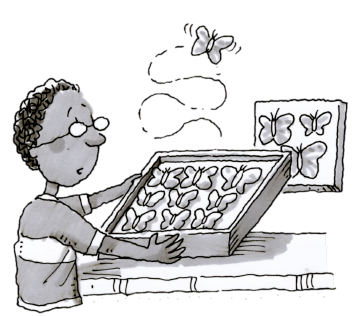### Home > CC2MN > Chapter 7 > Lesson 7.2.2 > Problem7-66

7-66.During this chapter, you will use your new solving skills to solve word problems. Think about and use the strategies you already have to answer the questions below.

1. Andy is $4$ years older than Eduardo. If Andy is $x$ years old, write an expression to represent Eduardo’s age.

If Eduardo is $4$ years younger than Andy, how could his age be represented if Andy is $x$ years old?

$x-4$

2. In Eduardo’s collection, the number of butterflies is $12$ more than twice the number of moths. If there are $x$ moths, write an expression to represent the number of butterflies he has.

If twice the number of moths, $x$, can be represented as $2x$, how can the number of butterflies be represented?